# HTML5 modern art style drawing generator written in 735 bytes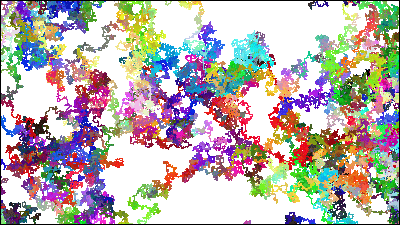MozillaFree MMORPG made with HTML 5 "BrowserQuest"Although it proves that it can be made with only HTML 5 if it is a certain degree of game, but what is possible what can be done if the source code is shortened as much as possible is this. The length of the source is 735 bytes, it is a generator that painting is painted like a modern art.

JS 1 k, 1 k demo submission 

Because it is 735 bytes of source full text, it is 735 characters (including one-byte space).

c.width=1000;c.height=1000;e=a.getImageData(0,0,1000,1000);d=e.data;k=1000;n=5;onkeydown=onkeyup=function(z){z=z.keyCode;k*=z==38?10:z==40?0.1:1;n+=z==37?-1:z==39?1:0;n=n<0?0:n;};g=120;l=120;m=Math;r=120;x=500;y=500;setInterval('j=k;while(j>0){i=m.random();if(i<0.25){x--;}else if(i<0.5){y--;}else if(i<0.75){x++;}else{y++;}i=m.random();if(i<0.166){r-=n;}else if(i<0.333){r+=n;}else if(i<0.5){g-=n;}else if(i<0.666){g+=n;}else if(i<0.833){l-=n;}else{l+=n;}if(x>999){x=0;}if(y>999){y=0;}if(x<0){x=999;}if(y<0){y=999;}if(r>255){r=255;}if(g>255){g=255;}if(l>255){l=255;}if(r<0){r=0;}if(g<0){g=0;}if(l<0){l=0;}d[(y*4000)+(x*4)]=r;d[(y*4000)+(x*4)+1]=g;d[(y*4000)+(x*4)+2]=l;d[(y*4000)+(x*4)+3]=255;j--;}e.data=d;a.putImageData(e,0,0);',1);

Drawing starts suddenly when you access the above URL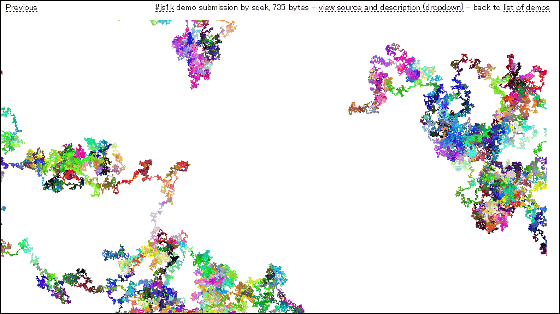If you leave it for a while it looks something like this.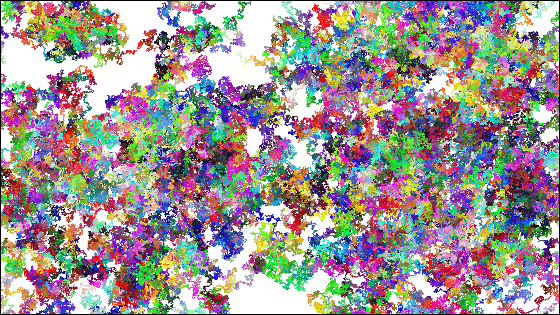Click "view source and description (dropdown)" to display the source code and description. When you press the cursor key up, the drawing speed is raised, when you press the bottom, the drawing speed is down.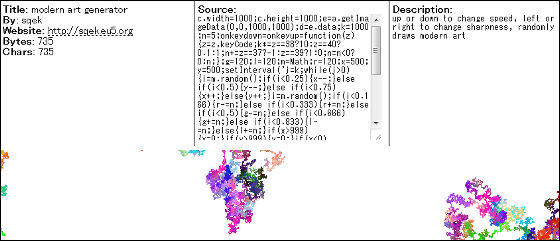When you press the left and right of the cursor key, the color change condition changes. In the case of the right, the same color is drawn for a longer time.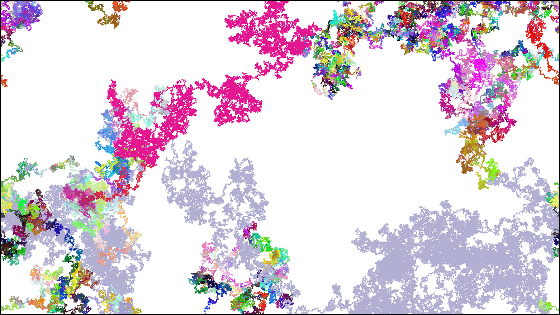When you press the left of the cursor key, the color changes faster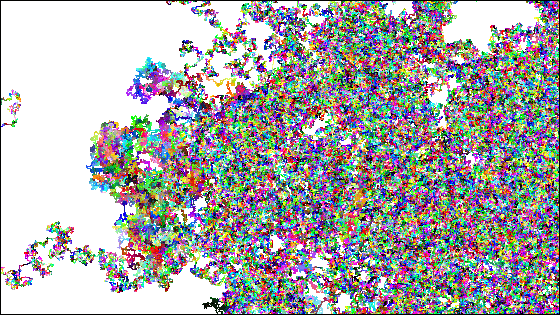Please be aware that scripts can not be processed completely if drawing speed is increased too much.

in Web Service, Posted by logc_nt